# The KDE Procedure

## Getting Started: KDE Procedure

The following example illustrates the basic features of PROC KDE. Assume that 1000 observations are simulated from a bivariate normal density with means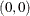, variances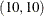, and covariance 9. The SAS DATA step to accomplish this is as follows:

data bivnormal;
seed = 1283470;
do i = 1 to 1000;
z1 = rannor(seed);
z2 = rannor(seed);
z3 = rannor(seed);
x = 3*z1+z2;
y = 3*z1+z3;
output;
end;
drop seed;
run;


The following statements request a bivariate kernel density estimate for the variables x and y, with contour and surface plots:

ods graphics on;
proc kde data=bivnormal;
bivar x y / plots=(contour surface);
run;
ods graphics off;


The contour plot and the surface plot of the estimate are displayed in Figure 48.1 and Figure 48.2, respectively. Note that the correlation of 0.9 in the original data results in oval-shaped contours. These graphs are produced by specifying the PLOTS= option in the BIVAR statement with ODS Graphics enabled. For general information about ODS Graphics, see Chapter 21: Statistical Graphics Using ODS. For specific information about the graphics available in the KDE procedure, see the section ODS Graphics.

Figure 48.1: Contour Plot of Estimated Density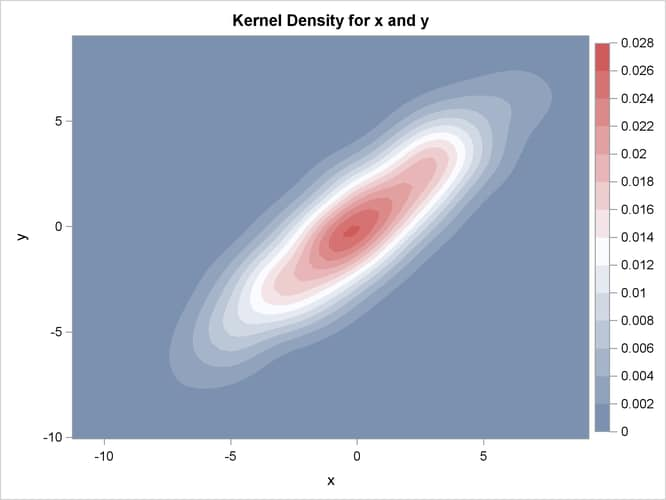Figure 48.2: Surface Plot of Estimated Density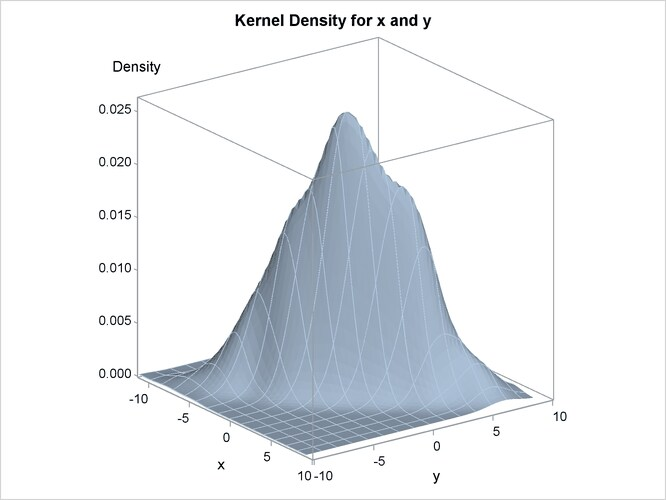The default output tables for this analysis are shown in Figure 48.3.

Figure 48.3: Default Bivariate Tables

The KDE Procedure

Inputs
Data Set WORK.BIVNORMAL
Number of Observations Used 1000
Variable 1 x
Variable 2 y
Bandwidth Method Simple Normal Reference

Controls
x y
Grid Points 60 60
Lower Grid Limit -11.25 -10.05
Upper Grid Limit 9.1436 9.0341
Bandwidth Multiplier 1 1

The Inputs table lists basic information about the density fit, including the input data set, the number of observations, and the variables. The bandwidth method is the technique used to select the amount of smoothing in the estimate. A simple normal reference rule is used for bivariate smoothing.

The Controls table lists the primary numbers controlling the kernel density fit. Here a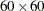grid is fit to the entire range of the data, and no adjustment is made to the default bandwidth.